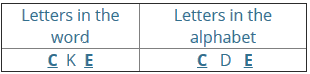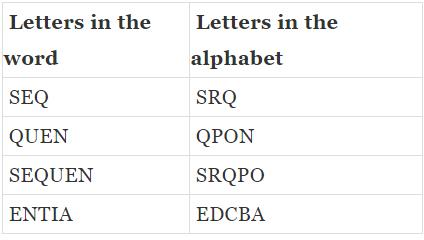Courses

# Alphabet MCQ 1

## 20 Questions MCQ Test Logical Reasoning for CLAT | Alphabet MCQ 1

Description
This mock test of Alphabet MCQ 1 for CLAT helps you for every CLAT entrance exam. This contains 20 Multiple Choice Questions for CLAT Alphabet MCQ 1 (mcq) to study with solutions a complete question bank. The solved questions answers in this Alphabet MCQ 1 quiz give you a good mix of easy questions and tough questions. CLAT students definitely take this Alphabet MCQ 1 exercise for a better result in the exam. You can find other Alphabet MCQ 1 extra questions, long questions & short questions for CLAT on EduRev as well by searching above.
QUESTION: 1

### If the first half of the English alphabet is reversed and then next portion of English alphabet is reversed so as 'A' takes the portion of 'M' and 'N' takes the portion of 'z' then which letter will be 6th to the left of 17th letter to the right of 7th leter from the left?

Solution:

6th to the left of 17th letter to the right of 7th letter from the left = 6th to the left of (17+7 =) 24th letter from the left = (24-6 =) 18th letter from the left.
Thus, we have to count from the left and we have to find out 18th letter from the left that falls into the second section of English alphabet.
Here, both the first half and the second half of the English alphabet have been reversed and we have to count from the left end, then the required letter can be found out using the formula given below. Suppose, the first and the second reversed sections of the English alphabet are α and  β respectively.Then,
The required letter = 2α+β+1−(required position of letter from left side)
Therefore, The required letter = 26 + 13 + 1 - 18 = 22 = V

QUESTION: 2

Solution:
1. C_A
2. RS

QUESTION: 3

### Q: A B C D E F G H I J K L M N O P Q R S T U V W X Y Z. Which letter in this alphabet is the eighth letter to the right of the letter which is tenth letter to the left of the last but one letter of the alphabet?

Solution:

In the given alphabet, last but one letter of alphabet is Y.

10th letter to the left of Y is O

8th letter to the right of O is W

QUESTION: 4

In the English Alphabet, which letter would be seventh to the left of fourteenth from right?

Solution:

Fourteenth from right(Z to M) = M
Seventh from left of M is F.

QUESTION: 5

In the English Alphabet, which letter would be tenth to the right of eighteenth from right?

Solution:

Starting from right means Z to A.
When the alphabets are counted from backward the total number of alphabets is considered as 27.
Eighteenth from right is I = 27-18 = 9
The tenth alphabet to the right of I will be S = 9+10 = 19

QUESTION: 6

In the English Alphabet, which letter would be 7th to the left of the letter S?

Solution:

The number of S in alphabet series is 19
Seventh to left of S = L

QUESTION: 7

In the English Alphabet, which letter would be fifteenth to the right of letter which is third to the left of letter J?

Solution:

When the alphabets are counted from backward the total number of alphabets is considered as 27.

The fifteeth letter to the right is 'L' according to 27 - 15 = 12 i.e. the 12th letter is 'L'.
The position of 'J' is 10 according to the English alphabet.
'L' position + 'J' position = 10 + 12 = 22 = V

QUESTION: 8

In a certain code 'PENCIL' is written as 'RCTAMJ' then in that code 'BROKEN' is written as :

Solution:

The first three letters of the word are reversed. Thus PENCIL becomes NEPCIL. Now add 4 to odd-positioned letters and subtract 2 from even-positioned ones.

Similarly, BROKEN becomes ORBKEN.
Then we do the calculations: O +4, R -2, B +4, K -2, E +4, N -2
Resulting word = SPFIIL

QUESTION: 9

In the English Alphabet which letter would be 7th to the right of 15th from left?

Solution:

Fifteen from left = O (15)
Seventh to right of O = 15+7 = 22
The 22th alphabet is V

QUESTION: 10

If the second half of the english alphabet is written in reverse order, which letter will be ninth of the right of the eighth letter from the left end?

Solution:

A,B,C,D,E,F,G,H,I,J,K,L,M,Z,Y,X,W,V,U,T,S,R,Q,P,O,N
Eighth letter from left end = H.
Ninth to the right of H = W.

QUESTION: 11

If second half of the following alphabet is written in reverse order then which letter will be 10th from left of 9th letter from the right end?
A B C D E F G H I J K L M N O P Q R S T U V W X Y Z

Solution:

A,B,C,D,E,F,G,H,I,J,K,L,M,Z,Y,X,W,V,U,T,S,R,Q,P,O,N
According to the arrangement
Ninth letter from the right end = V
Tenth letter to left of V = H.

QUESTION: 12

Which letter is exactly midway between H and S in the alphabet series?

Solution:

There are ten letters between H and S and as such there is no letter which lies in the middle

QUESTION: 13

How many pairs of letters are there in the word BUCKET which has as many letters between them in the word as in the alphabet?

Solution:QUESTION: 14

Which of the following will be the changed form of the word OBLIQUE when the word is written again by substituting each vowel by the 2nd letter following it in the English alphabet and each consonant is substituted by the 3rd letter following it in the English alphabet?

Solution:

The Given word is OBLIQUE.

After making necessary changes that are all vowels are substituted by the next second letter and all consonants are substituted by the next third letter in the English alphabet.

Thus, the resulting word is QEOKTWG.

QUESTION: 15

If a meaningful word can be formed using the five letters NWROD each only once, then the fourth letter of that word is your answer. If more than one such word can be formed then Y is your answer, and if no such word can be formed then 'Z' is your answer.

Solution:

Here specified letters are N,W,R,O and D. The meaningful word made from these letters is DROWN.
Thus, the answer is W the fourth letter of the only word possible.

QUESTION: 16

If it is possible to make a meaningful word with the first, the fourth, the seventh and the eleventh letters of the word "INTERPRETATION', which of the following will be third letter of that word ? If more than one such word can be made, give M as the answer and if no such word can be made, give X as the answer.

Solution:

The first, fourth, seventh and eleventh letters of the word INTERPRETATION are I, E, R and T respectively. The words formed are TIER, RITE and TIRE.

Thus M is the answer as more than one word can be formed.

QUESTION: 17

If the alphabet is written in the reverse order and every alternate letter starting with Y is dropped, which letter will be exactly in the middle of the remaining, letters of the alphabet.

Solution:

Canceling every second letter after reversing the alphabet the series becomes: Z X V T R P N L J H F D B
Thus, the middle letter is N

QUESTION: 18

If it is possible to make a meaningful word with the 2nd, the 3rd, the 4th and the 6th letter of the word STRATEGIC; then which of the following will be the 2nd letter of the word? If no such word can be made, mark ‘X’ as your answer. If more than one such word can be made, mark ‘M’ as your answer.

Solution:

The 2nd, the 3rd, the 4th and the 6th letter of the word STRATEGIC are T,R,A and E respectively.
The words can be RATE and TEAR.
Thus M is the answer as more than one word can be formed.

QUESTION: 19

A B C D E F G H I J K L M N O P Q R S T U V W X Y Z

If the first half of the above alphabets are written in the reverse order, which letter will be exactly middle between the 9th letter from the left and the 10th letter from the right end?

Solution:

The arrangement will be: M L K J I H G F E D C B A N O P Q R S T U V W X Y Z
9th letter from left = E.
10th letter from right = Q
A lies exactly in middle between E and Q

QUESTION: 20

How many pairs of letters are there in the word 'SEQUENTIAL' which have as many letters between them as are in the alphabet?

Solution: